Deutsche VersionConversion: Voltage V to Level, dB, dBu, dBV,
and power level
dBm with impedance value ●

Usually we measure AC voltage V as voltsRMS.
The RMS value of a set of values is the square root of the
arithmetic mean (average) of the squares of the original values.

 Voltage V volts (Root Mean Square = RMS) ↓ Voltage level L Lu dBu Reference: 0.775 V LV dBV Reference: 1 V Power level L Not used for audio! L dBm > > > ref. 1 mW at ohms
The index u at decibel (dB) means unloaded source, V (volt). Some say: The "u" in dBu
implies also that the load impedance is unspecified, or unterminated and is likely to be high.
The index m at dB (decibel) means 1 milliwatt (power) as reference value for 0 dB.

If the reverse conversion dBu, or dBV to volts is needed, go to the converter at the bottom.

● What is dBu? That has nothing to do with power.
A logarithmic voltage ratio with a reference voltage of
V0= 0.7746 volt ≡ 0 dBu

● What is dBV?
A logarithmic voltage ratio with a reference voltage of
V0 = 1.0000 volt ≡ 0 dBV
The max. domestic recording level of −10 dBV means 0.3162 volts, that is −7.78 dBu

 Level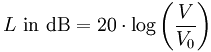Voltage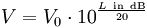● What is dBm? That has nothing to do with voltage.
A logarithmic ratio with a reference power of
P0 = 1.000 milliwatt ≡ 0 dBm.
The pseudo unit "dBm" is not used in audio and in sound recording.
With the known impedance value you can convert voltage V to level dBm (power) and vice versa.
Often an impedance of 600 ohms or 50 ohms is assumed.

For radio frequency systems (RF), impedance matching or power matching is absolutely essential.

 Level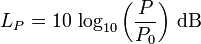Power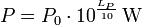The dBm calculator for impedance matching
or power matching

 In sound engineering there is no Power matching or Impedance matching. In audio we use only Voltage bridging or high Impedance bridging.

Enter two values, the third value will be calculated.
Voltage
V, level L, and impedance Z.

 Voltage V V RMS Power level L dBm re 1 mW Impedance Z Ω

dBm indicates that the reference power is P0 = 1 milliwatt = 0.001 watt ≡ 0 dBm
 To use the calculator, simply enter a value. The calculator works in both directions of the ↔ sign.

 Electric power (phone) P:  watts ↔ Electric Power level LP: dBmReference power P0 = 1 milliwatt (mW) = 0.001 W ≡ 0 dBm

Telephone lines need an input impedance and
an output impedance of 600 ohms for
impedance matching (power matching).

 Reference voltage at 600 ohms – 1 mW according to 0 dBmReference voltage at 50 Ohm – 1 mW according to 0 dBm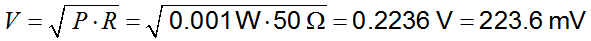dBu is a voltage standard where "zero" (as in zero dB on your meters) is considered to be 0.775 volts and +4 dBu is therefore 1.23 volts. dBm is a power measurement (the "m" stands for milliwatts), where +4 also happens to be 1.23 volts if the load impedance is 600 ohms. In many cases (and this is "old school" audio) a 600 ohm load can be assumed, however, in modern day interconnects input impedances are usually much higher, which kind of renders the dBm standard useless. This is one reason why most gear is rated in dBu, or in dBV (another voltage standard).

Impedance matching Z2 = Z1 for telephone (phone) lines
Transformer matched 600 ohm phone transmission line

 Telephone line amplifier 1 → Telephone line amplifier 2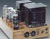Source impedance Z2 ohms    |      Load impedance Z1 ohms Voltage damping by load Δ L dB Damping factor DF = Zin / Zout
 ● Definition of dBm   dBm is defined as power ratio in decibel (dB) referenced to one milliwatt (mW). It is an abbreviation for dB with respect to 1 mW and the "m" in dBm stands for milliwatt.   dBm is different from dB. dBm represents absolute power, whereas in audio engineering the decibel is usually a voltage ratio of two values and is used then to represent gain or attenuation of an audio amplifier, or an audio damping pad.   If you use modern audio equipment in sound engineering, calculations used at typically 600 ohms impedance for telephone lines or 50 ohmsfor antennas, are not correct. Matching impedance for power is not used in the field of audio. You will only use voltage bridging or impedance bridging Zout << Zin (Zsource << Zload)   Here are adequate calculators for audio voltage and voltage levels: